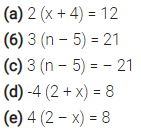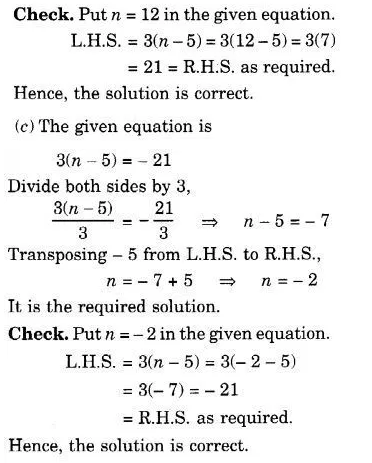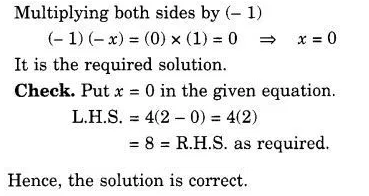# Solve the following equations : (a) 2 (x + 4) = 12 (6) 3 (n – 5) = 21

Solve the following equations